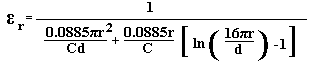# Relative Dielectric Constant Calculator for a Circular Capacitor

The relative dielectric constant (εr) of the dielectric in a parallel plate, circular capacitor can be calculated using the following formula.Where r is the Radius in cm

C is the Capacitance in pico Farads

d is the Dielectric thickness in cm

 Enter the value of the capacitor: pF Enter the radius of the circle : cm Enter the thickness of the dielectric : mm Relative Dielectric Constant (εr):

This calculator uses Visual Basic and will work in Internet Explorer and other browsers with Visual Basic plug-ins. For more information see About our calculators. A further simple Relative Dielectric Constant Calculator is available in JavaScript.

The calculator uses a derivation of Kirchhoff's formula for a circular capacitor and is intended for use with the Accurate Method for Measuring Relative Dielectric Constant of a PCB Using a Vector Network Analyser

This calculator is provided free by Chemandy Electronics in order to promote the FLEXI-BOX